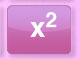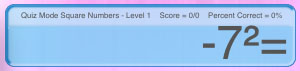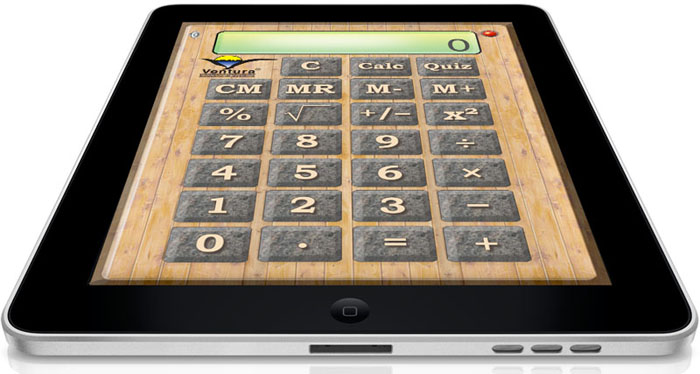An Elementary Calculator that Quizzes Kids on the Types of Problems that They Shouldn't Need to Use a Calculator to Solve!
 Now also available for Apple TV. Suddenly, it OK for kids to sit in front of the TV and do their homework. QuizCalc is a calculator that actually helps kids improve math skills. Tap the quiz button and instantly QuizCalc starts generating 8 different types of math problems - everything from addition to finding percentages. QuizCalc has two levels of difficulty and is perfect for families with one or more elementary age children. Make the time kids spend in front of the TV productive with QuizCalc!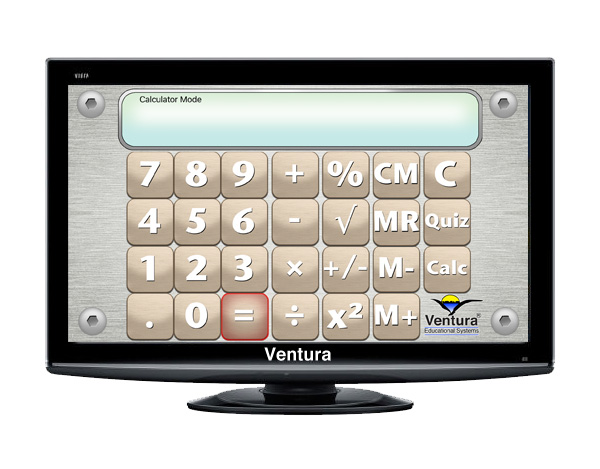For iPad, iPhone, iPod and now Apple TV...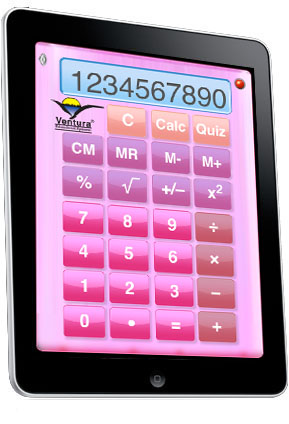Check out our Hands-On Math Series! QuizCalc is a basic calculator perfect for elementary age students with percent, square root, integer, power and memory ( +, – , C & R ) functions. Tap the Quiz button and QuizCalc becomes a math quiz generator providing students with a great way to practice math facts and concepts. Here are a few of the ways you will use QuizCalc everyday with your elementary age students: • Use QuizCalc just like any other calculator to help with math studies. •Tap the Quiz button and QuizCalc shifts into a game where students practice arithmetic facts. •Use QuizCalc everyday to improve scores on 8 different randomly generated quizzes at 2 levels.

QuizCalc Calculator Function

Tap the Calculator button to switch QuizCalc into Calculator Mode. In Calculator Mode, QuizCalc works just like any other algebraic calculator. Here are some examples of how to do some basic calculations:

 Operation Enter Display addition 1 + 2 = 3 subtraction 10 - 7 = 3 multiplication 5 × 8 = 40 division 60 ÷ 12 = 5 percent 100 + 10 % = 110 square root 81 √ 9 integer 12 +/- -12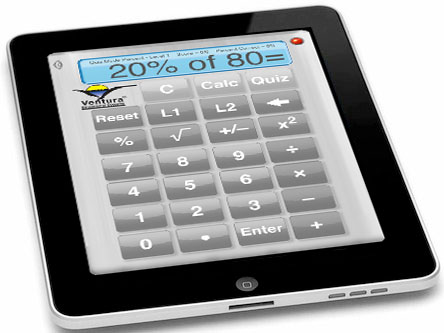\$1.99

QuizCalc Mental Math Quiz Function

In addition to functioning as a regular calculator, QuizCalc provides practice in solving the types of problems for which they shouldn't need to use a calculator. students with practice in the following mental math areas:

 Topic Level Math Objectives Addition of Whole Numbers 1 Addition Facts with Numbers from 0 to 10. 2 Addition Facts with Numbers from 10 to 40. Subtraction of Whole Numbers 1 Subtraction Facts with Numbers from 0 to 20. 2 Subtraction Facts with Numbers from 10 to 60. Multiplication of Whole Numbers 1 Multiplication Facts with Numbers from 0 to 10. 2 Multiplication Facts with Numbers from 0 to 20. Division of Whole Numbers 1 Division Facts with Divisors from 1 to 10. 2 Division Facts with Divisors from 1 to 20. Percents 1 Problems with Percents from 10 to 100. 2 Problems with Percents from 10 to 200. Roots of Perfect Squares 1 Find Roots from 1 to 10. 2 Find Roots from 5 to 12. Addition of Integers 1 Numbers from -5 to +5. 2 Numbers from -10 to +10. Perfect Squares 1 Find the Square of Numbers from 1 to 10. 2 Find the Square of Numbers from -10 to 10.
 Enjoy using QuizCalc on your iPhone, iPod or iPad. In addtion to being an easy-to-use calculator, it's a great way to practice math skills. Parents and teachers will like the score keeping function that reports how well the student is doing in each of the skill areas. The score shows how many problems were attempted, then number correct and a percentage.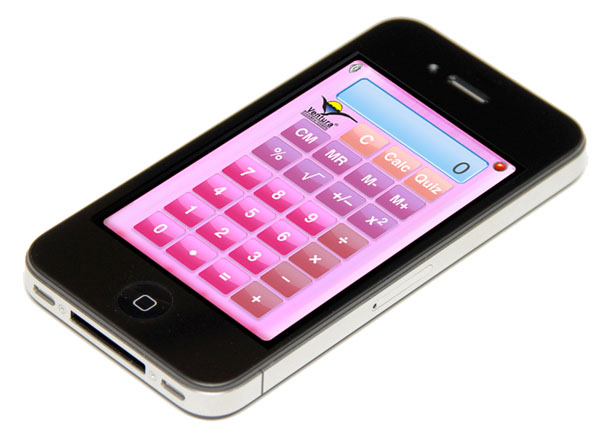Sample Problem Displays

In Quiz Mode, QuizCalc randomly generates problems at two levels. The score tally and percent correct is shown in the calculator display. Visual and auditory feedback responds after student enters each answer.

 Press this Key Problem Sets Level 1 - Addition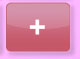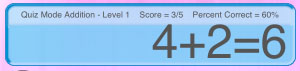Level 2 - Addition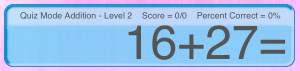Level 1 - Times Tables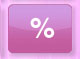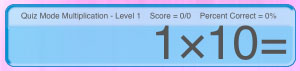Level 2 - Times Tables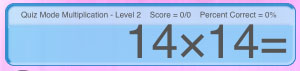Level 1 - Integer Addition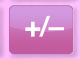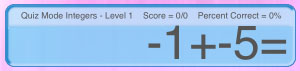Level 1 - Percent Problems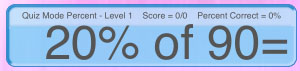Level 1 - Square a Number